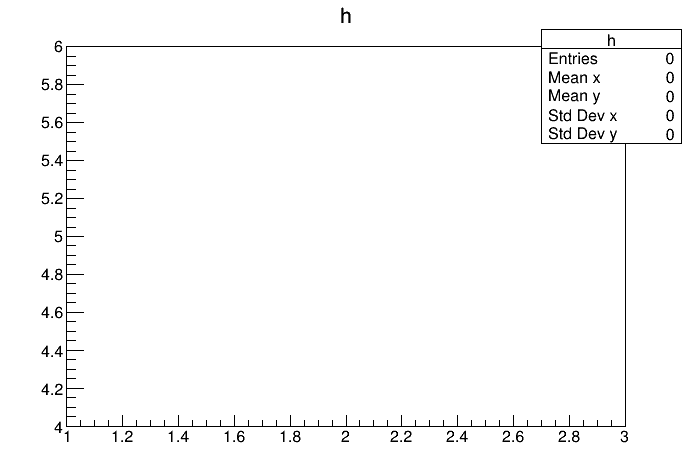# [Solved] TH2D empty using the constructor with arrays

_ROOT Version:6.18/00
Platform: x86_64-centos7-gcc8-opt
Compiler: gcc8

Dear all,

Here is a minimal non working example of the issue:

In : h1 = np.array([1,2,3])
In : h2 = np.array([4,5,6])
In : from array import array
In : h = r.TH2D(“h”, “h”, len(h1) -1, array(‘d’, h1), len(h2) -1, array(‘d’, h2))
In : h.Draw()
In : h.GetBinContent(10)
Out: 0.0As you can see, the TH2 is empty.

This little test was done using Python 2.7.16

This used to work in previous ROOT versions. It was even possible to build the TH2 using numpy arrays (without transforming them to C-like arrays).

If I try to use plain numpy arrays:
In : h1 = np.array([1,2,3,4])
In : h2 = np.array([10,20,30,40])
In : h = r.TH2D(“h”, “h”, len(h1) -1, h1, len(h2) -1, h2)
In : h.Draw()
Info in TCanvas::MakeDefCanvas: created default TCanvas with name c1
In : Error in TCanvas::Range: illegal world coordinates range: x1=0.000000, y1=0.000000, x2=0.000000, y2=0.000000
Error in TCanvas::RangeAxis: illegal axis coordinates range: xmin=0.000000, ymin=0.000000, xmax=0.000000, ymax=0.000000`

Thx,
Fabrice

Hi,
I see the same result in the C++ equivalent:

``````{
double h1 = { 1., 2., 3. };
double h2 = { 4., 5., 6. };
TH2D *h = new TH2D("h", "h", 2, h1, 2, h2);
h->Draw()
}
``````

It does take the values we pass into account since it sets the x and y axis accordingly.
Side note: in python you can still use numpy arrays, just make sure the element type is double:

``````import ROOT as r
import numpy as np
h1 = np.array([1.,2.,3.])
h2 = np.array([4.,5.,6.])
h = r.TH2D("h", "h", len(h1) -1, h1, len(h2) -1, h2)
h.Draw()
``````

Hi,
Thx for this useful information. So I guess this is a plain ROOT bug, not related to the interface with python. I removed the python flag from the topic.

Hi,
It is not a bug, it is the expected behaviour of the method.

If you check the documentation for the equivalent constructor in `TH1`:
https://root.cern.ch/doc/master/classTH1.html#a3ba472a2a1e853307c8368ddba833637

The arrays you pass just set the low edges for the bins, they do not set the content. You need to fill the histogram to see the bins appearing in the plot.

Ah yes of course, sorry for being silly, this is just setting the bins !

@fadesse I am not sure this solves the problem we have. We fill the histogram and we persist it to disk. Then we read it back and the emptiness of the histo is still present although the GetBinContent returns non 0 values.

Hi Renato,

Can you share a reproducer for the code you use to persist the histogram to disk and read it back? If you fill it before persisting, it should have the filled entries when reading back.

Cheers,
Enric

We are using something like:

``````h = r.TH2D(name, title, len(self.edges) - 1, self.edges, len(self.edges) - 1, self.edges)
for i in range(h.GetNbinsX()):
for j in range(h.GetNbinsY()):
h.SetBinContent(i + 1, j + 1, self.histo[i, j].n)
if isSumw2:
h.SetBinError(i + 1, j + 1, self.histo[i, j].s)

file = r.TFile(outfile + ".root", "RECREATE")
h.Write()
del h
file.Close()
``````

where self.edged[i] are numpy arrays (I guess these are int, and this is what is causing the issues).
Later on, opening and drawing interactively the histogram in ROOT, we get stuff like

``````Error in TCanvas::Range: illegal world coordinates range: x1=0.000000, y1=0.000000, x2=0.000000, y2=0.000000
Error in TCanvas::RangeAxis: illegal axis coordinates range: xmin=0.000000, ymin=0.000000, xmax=0.000000, ymax=0.000000`
``````

Although when checking with GetBinCOntent, we see that content of the histogram.

I would try with the double-typed numpy arrays.

Also I would create the file before creating the histograms.

On the other hand, why do you set `nbins` to `len(edges) - 1` instead of the number of low edges (i.e. `len(edges)`)?

Yes indeed, it works fine either with double-typed numpy arrays or using the array package to transform the numpy array to a double array.

The len(edges) -1 is because the number of bins is equal to the number of limits -1 (eg: [1,2,3] -> 2 bins).

Thx for your help, I think the issue is solvedI guess in previous versions, ROOT was more permissive and somehow also accepted an array of int for the bins, but now it has to be an array of double.

This topic was automatically closed 14 days after the last reply. New replies are no longer allowed.